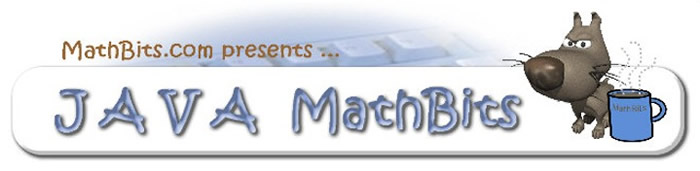Beginning Java Programming
with a Mathematical Flair!

In over 38 states, computer science can be used toward meeting high school mathematics graduation requirements (with a few states extending this to include science requirements as well). In 2014, New York state announced the use of courses in Computer Science to meet the requirements for a Regents or Local Diploma in mathematics or science. A mention was made of an integrated course combining technology with mathematics and/or science as a follow-up course for students who have passed the required state assessment.

 Have an account?
With the idea of offering a programming course with a mathematical flair, we have updated our full year Beginning Java course to contain more mathematical concepts, and to run under a "free" IDE which will be financially friendlier for school districts and students. This new curriculum will be using Eclipse as the IDE, with an emphasis on Java application programming.
 We will be using: Java SE 8 with JDK 1.8 (Java 8). Eclipse Version: Neon Release (4.6) http://www.eclipse.org
This course is not aimed at the AP Computer Science A Examination. Its goals are to give students a solid foundation in the principles of programming, an opportunity to revisit some mathematical concepts, and an experience that is challenging yet enjoyable. Maybe, even fun!!
This subscription is a creative collection of over 342 resource materials and 32 interactive on-line activities.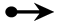How to Subscribe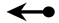Profits are donated to our local animal shelters and to support JavaBitsNotebook.com.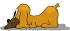Attention Past Java CD Subscribers!

 Full Year Course Curriculum (aligns with "free" on-line lessons found at http://JavaBitsNotebook.com.) The Java MathBits Subscription includes: • content aligning with the Units of Study shown below. Each content topic includes: • hard copy handouts of all lessons appearing on JavaBitsNotebook. • in-class lab programming problems to emphasize the latest concepts. • programming projects to allow students to demonstrate their skills. • sample .java files provided as demo solutions for all projects. • assignment worksheets. • quizzes (hardcopy for certain units). • quizzes (on-line for all units) • Unit Tests. • calendars (at two pacing levels) • classroom group activities. • a wide variety of on-line interactive games/activities. Furthermore: • Tri-fold Class Information Brochure • Tri-fold Brochure for Using Eclipse Software (Quick Reference) • Parent Night Slide Presentation • Course Outline • Yearly layout planning calendars (at two pacing levels) • Mid-Year review and examination. • Final Examination • ALL materials/activities/games are iPad (touch screen) compatible. • All answer keys are provided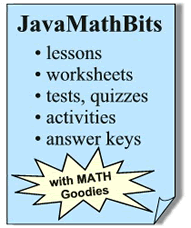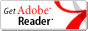Nearly all files are in .pdf (which requires free Acrobat Reader) or .java format.

 Check out the connection between mathematics and computer programming, along with the general math topics per JavaMathBits Units of Study.

The table below shows the topical outlines with the mathematical influences found in each unit of study.

Units of Study

UNIT Topic:
Math Influence: (by specific topics)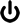Unit 1:  Introduction
Number Bases Conversions (Bases 10, 2, 8, 16), Working with Integer Exponents, Writing Explicit Directions, Interpreting Descriptions & Directions, Problem solvingUnit 2:  Data Basics
Integers and Rationals, Scientific Notation, Number Properties, Order of Operations, Modulus (Remainder), Computer Equations versus Mathematical Equations, Number digits, Circles Area & Circumference, Triangle Area, Money & Coins, Volume, Pythagorean Triples, Measurement conversions, Rounding, Division by Zero, Organization of Concepts, Problem solvingUnit 3:  Conditionals
Trichotomy, And/Or/Not Logic, Truth Tables, Mention of DeMorgan's Laws, Operator Precedence, logical comparison to graphing calculators, Determining Costs, Determining odd/even, Determining types of triangles, Determining sides of triangles, Measurement conversions, Sudoku Reasoning, Problem solving.Unit 4:  Looping
Representing odd/even integers, Factorial, Prime numbers, Quadratic Formula, Fibonacci Sequence, Code Process to Convert Binary to Decimal, Powers of 2, Sum of Digits, Division by Zero, Modulus Operator, Averaging, Repetitive Process, Incremental Counting, Nesting Process, Problem Solving, Logical Reasoning.Unit 5:  Methods
Square Feet, Triangular Numbers, Harmonic Mean, Squares, Cubes, Determining Cost & Tax, Series, Circle Area, Function Input/Output, Linear Regression, Discriminant, Logical Operators, Determining Minimum Value by Comparison, Geometric Designs, Right Triangles, Rectangles, Isosceles Triangles, Problem Solving, Reasoning.MidYear Review/Exam
OPTIONAL: To be used for testing for a one semester course, for schools mandating mid-year exams, for simply review, or not at all. Depends upon your needs and time frame.Unit 6:  Library Methods
Rounding techniques, Absolute value, Square roots, Exponents, Maximum/minimum, Area/circumference of circles, Exponential growth, Rolling Dice, Experimental Probability, Dealing with Percentages, Right tingles, Pythagorean Theorem, Trigonometry, Find angle in a right triangle, Convert radians to degrees, Random Generation Formulas, Dealing with time, Problem Solving, Reasoning.Unit 7: Files
Random number formulas, Average, Gross pay, Determining odd/even, Division by zero, Statistical Analysis, Mean, Sum of Differences Squared, Variance, Standard DeviationUnit 8:  Arrays
Designation of zero as odd/even/neither/positive/negative, Proper Subscripting, Showing odd/even, Average, Cost, Rounding, Reverse thinking, Number comparisons, Percentage of, Sequential searching, Binary searching, Matrices, Magic squares, Random numbers, Number analysis, Maximum/minimum, Range, Median, Variance, Standard deviation, Data manipulation, Histograms, GPAs, Processing Fees, Sorting, Palindromes, Problem Solving, Reasoning.Unit 9:  Graphics Drawing
Algebraic expressions, Properties of geometric figures, Working on coordinate axes, Mathematics of Color, Similar figures, Central angles and arcs, Unit circle, Random numbers, Formula development in animation, Storage arrays, Recursive reasoning, Logical reasoning, Problem Solving.Unit 10: GUI
Perfect squares, Prime numbers, Working with GPA, Determining lengths of sides of triangle, Pythagorean Theorem to test for right triangle, Determining BMI, Random number generation, Probability die, Divisibility by 3, Unit conversions, Sigma notation for series, Types of Triangles.Final Project / Exam
To be used for testing a full year course or the second semester of a two semester course.
 Interactive Materials
 Teaching Strategies

Math Influence: The mathematical topics are appropriate for students having completed, or are currently enrolled in, Algebra 1. The concept of working with variables is expected. Topics that go beyond the scope of Algebra 1 are explained within the context of the problem. While not required, teachers may take the opportunity to briefly discuss such topics.

Programming Difficulty Level: This curriculum was originally designed as the first year in a two year high school program leading to the old Advanced Placement AB Examination in Computer Science (Java). The course now includes nearly all of the materials (updated) from that original CD course, with the addition of more mathematical applications, more on-line activities, new organizational calendars at varying paces, and new coordination with the free IDE Eclipse. Teachers will find an abundant amount of materials from which to "pick-and-choose" at difficulty levels ranging from easy to challenging.

Your Experience Teaching Programming: If you are new to teaching programming, or an old pro, we have you covered.
• The "lesson" pages at JavaBitsNotebook help you coordinate what materials need to be addressed. You can project the pages and use them as talking points.
• The worksheets, assignments, and projects coordinate with the lesson concepts.
• The classroom activities add "flare" to the daily routine.
• The on-line activities and quizzes keep your course relevant and "connected".
• The tests and quizzes measure students' understanding of the concepts. The difficulty range of the questions may vary, but there are no challenging questions beyond the grasp of a well prepared student.

Our Experience Teaching Programming: In addition to being career mathematics teachers, both Fred and Donna have taught high school computer programming (BASIC, Pascal, C++, Java) including teacher workshop offerings (TI-Calculator Programming, TI-Robot Programming), with Fred also teaching Computer Science at the college level.How to SubscribeProfits are donated to our local animal shelters and to support JavaBitsNotebook.com.